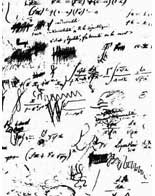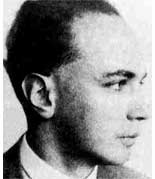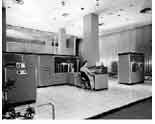# Tag: simplesLast time we have seen that in order to classify all
non-commutative $l$-points one needs to control the finite
dimensional simple algebras having as their center a finite
dimensional field-extension of $l$. We have seen that the equivalence
classes of simple algebras with the same center $L$ form an Abelian
group, the
Brauer group. The calculation of Brauer groups
is best done using
Galois-cohomology. As an aside :
Evariste Galois was one of the more tragic figures in the history of
mathematics, he died at the age of 20 as a result of a duel. There is
a whole site the Evariste Galois archive dedicated to his
work.

But let us return to a simple algebra $T$ over the
field $L$ which we have seen to be of the form $M(k,S)$, full
matrices over a division algebra $S$. We know that the dimension of
$S$ over $L$ is a square, say $n^2$, and it can be shown that all
maximal commutative subfields of $S$ have dimension n over $L$.
In this way one can view a simple algebra as a bag containing all
sorts of degree n extensions of its center. All these maximal
subfields are also splitting fields for $S$, meaning that
if you tensor $S$ with one of them, say $M$, one obtains full nxn
matrices $M(n,M)$. Among this collection there is at least one
separable field but for a long time it was an open question
whether the collection of all maximal commutative subfields also
contains a Galois-extension of $L$. If this is the case, then
one could describe the division algebra $S$ as a crossed
product
. It was known for some time that there is always a simple
algebra $S’$ equivalent to $S$ which is a crossed product (usually
corresponding to a different number n’), that is, all elements of
the Brauer group can be represented by crossed products. It came as a
surprise when S.A. Amitsur in 1972 came up with examples of
non-crossed product division algebras, that is, division algebras $D$
such that none of its maximal commutative subfields is a Galois
extension of the center. His examples were generic
division algebras
$D(n)$. To define $D(n)$ take two generic
nxn matrices
, that is, nxn matrices A and B such that all its
entries are algebraically independent over $L$ and consider the
$L$-subalgebra generated by A and B in the full nxn matrixring over the
field $F$ generated by all entries of A and B. Somewhat surprisingly,
one can show that this subalgebra is a domain and inverting all its
central elements (which, again, is somewhat of a surprise that
there are lots of them apart from elements of $L$, the so called
central polynomials) one obtains the division algebra $D(n)$ with
center $F(n)$ which has trancendence degree n^2 1 over $L$. By the
way, it is still unknown (apart from some low n cases) whether $F(n)$
is purely trancendental over $L$. Now, utilising the generic
nature of $D(n)$, Amitsur was able to prove that when $L=Q$, the
field of rational numbers, $D(n)$ cannot be a crossed product unless
$n=2^s p_1…p_k$ with the p_i prime numbers and s at most 2. So, for
example $D(8)$ is not a crossed product.

One can then
ask whether any division algebra $S$, of dimension n^2 over $L$, is a
crossed whenever n is squarefree. Even teh simplest case, when n is a
prime number is not known unless p=2 or 3. This shows how little we do
know about finite dimensional division algebras : nobody knows
whether a division algebra of dimension 25 contains a maximal
cyclic subfield (the main problem in deciding this type of
problems is that we know so few methods to construct division
algebras; either they are constructed quite explicitly as a crossed
product or otherwise they are constructed by some generic construction
but then it is very hard to make explicit calculations with
them).Non-commutative geometry seems pretty trivial compared
to commutative geometry : there are just two types of manifolds,
points and curves. However, nobody knows how to start classifying
these non-commutative curves. I do have a conjecture that any
non-commutative curve can (up to non-commutative birationality) be
constructed from hereditary orders over commutative curves
by universal methods but I’ll try to explain that another
time.

On the other hand, non-commutative points
have been classified (at least in principle) for at least 50
years over an arbitrary basefield $l$. non-commutative
$l$-points $P$ is an $l$-algebra such that its double
$d(P) = P \\otimes P^o$ ( where $P^o$ is the opposite algebra,
that is with the reverse multiplication) has an element$c=\\sum_i a_i \\otimes b_i with \\sum_i a_ib_i = 1 (in$P$)$ and such that for
all p in $P$ we have that $(1 \\otimes a).c = (a \\otimes 1).c$ For
people of my generation, c is called a separability idempotent
and $P$ itself is called a separable $l$-algebra.
Examples of $l$-points include direct sums of full matrixrings
(of varying sizes) over $l$ or group-algebras $lG$ for $G$ a
finite group of n elements where n is invertible in $l$. Hence, in
particular, the group-algebra $lG$ of a p-group $G$ over a field $l$
of characteristic p is a non-commutative singular point and
modular representation theory (a theory build almost single
handed by
Richard Brauer) can be viewed as
the methods needed to resolve this singularity. Brauer’s name is
still mentioned a lot in modular representation theory, but another
of his inventions, the Brauer group, seems to be hardly known
among youngsters.

Still, it is a crucial tool
in classifying all non-commutative $l$-points. The algebraic
structure of an $l$-point $P$ is as follows : $$P = S_1 + S_2 + … + S_k$$ where each S_i is a simple algebra (that is, it
contains no proper twosided ideals), finite dimensional over
its center $l_i$ which is in its turn a finite dimensional
separable field extension of $l$. So we need to know all
simple algebras $S$, finite dimensional over their center $L$ which
is any finite dimensional separable field extension of $l$. The
algebraic structure of such an $S$ is of the form$$S = M(a,D)$$ that
is, full axa matrices with entries in $D$ where $D$ is a
skew-field (or some say, a division algebra) with
center $L$. The $L$-dimension of such a $D$ is always a square,
say b^2, so that the $L$-dimension of $S$ itself is also a square
a^2b^2. There are usually plenty such division algebras, the simplest
examples being quaternion algebras. Let p and q be two
non-zero elements of $L$ such that the conic $C : X^2-pY^2-bZ^2 = 0$ has no $L$-points in the projective $L$-plane, then the
algebra$D=(p,q)_2 = L.1 + L.i + L.j + L.ij where i^2=p, j^2=q and ji=-ij$ is a four-dimensional skew-field over $L$. Brauer’s idea to
classify all simple $L$-algebras was to associate a group to them,
the Brauer group, $Br(L)$. Its elements are equivalence
classes
of simple algebras where two simple algebras $S$ and
$S’$ are equivalent if and only if$M(m,S) = M(n,S’)$ for some sizes
m and n. Multiplication on these classes in induced by
the tensor-product (over $L$) as $S_1 \\otimes S_2$ is again a simple
$L$-algebra if $S_1$ and $S_2$ are. The Brauer group $Br(L)$ is an
Abelian torsion group and if we know its structure we know all
$L$-simple algebras so if we know $Br(L)$ for all finite dimensional
separable extensions $L$ of $l$ we have a full classification of
all non-commutative $l$-points.

Here are some examples
of Brauer groups : if $L$ is algebraically closed (or separable
closed), then $Br(L)=0$ so in particular, if $l$ is algebraically
closed, then the only non-commutative points are sums of matrix rings.
If $R$ is the field of real numbers, then $Br(R) = Z/2Z$ generated by
the Hamilton quaternion algebra (-1,-1)_2. If $L$ is a complete
valued number field, then $Br(L)=Q/Z$ which allows to describe also
the Brauer group of a number field in terms of its places. Brauer groups
of function fields of (commutative) varieties over an algebraically
closed basefield is usually huge but there is one noteworthy
exception $Tsen’s theorem$ which states that $Br(L)=0$ if $L$ is the
function field of a curve C over an algebraically closed field. In 1982
Merkurjev and Suslin proved a marvelous result about generators of
$Br(L)$ whenever $L$ is large enough to contain all primitive roots
of unity. They showed, in present day lingo, that $Br(L)$
is generated by non-commutative points of the quantum-planes
over $L$ at roots of unity. That is, it is generated by cyclic
algebras
of the form$(p,q)_n = L \\< X,Y>/(X^n=p,Y^n=q,YX=zXY)$where z is an n-th primitive root of
unity. Next time we will recall some basic results on the relation
between the Brauer group and Galois cohomology.Never
ever tell an ICT-aware person that you want to try to set up a
home-network before you understand all 65536 port-numbers and their corresponding
protocols. Here is what happened to me this week. Jan Adriaenssens returned from an extended vacation in New Zealand and I told him
about my problems with trying to set up WebDAV securely. He
stared at me with that look that teenage children have if they
find out their parents dont know how to handle the simplest things on a
mobile such as saving a number, writing an SMS let alone use the
dictionary… and asked ‘now why would you want to do that??? I just
use AppleTalk to connect to my computer securely’. Now I’m not such a
fool that I didnt try this out but I didnt manage to get matrix
mounted on my Desktop. ‘Oh, but thats probably because of the
firewall’ Jan said ‘just send an email to Peter (the guy running the
defenses here) and ask him to open up ports 548 and
427…’ And sure enough five minutes later the problem was
solved and I could trow my WebDAV-plans in the dustbin (although, I
think Ive found a use for WebDAV but will keep this a bit longer to
myself until I checked it out). If you think that was the end of it,
think twice. Never ever point an ICT-professional to your
computer. They then start looking at its firewall-logs and find all
sorts of things such as : ‘I noticed that traffic from port 53
was dropped to the firewall, could it be that you configured the
firewall as DNS-server. If this is the case, you better remove it and it
will increase your network-speed, I think.’ And sure enough that
IP-address was set on my machine as one of two possibilities for the
DNS-server so I quickly removed it and in the process thought that maybe
I should also remove the other one so I did send Peter another email
asking whether that was ok. It turned out that the second IP address was
the genuine DNS-server so I got a sec answer back ‘You better leave
this as it is otherwise not much will work…’ Oh, shame, shame eternal
shame on me!

My only defense is that I still belong
to what I would call the cpu 2 generation (I’m a few years too
old to belong to the more computer-aware generation X). When I
started out doing research in 1980 the single most important command was

cpu 2

which you had to type before you could run any program.
By typing this you asked to be given 2 minutes of central processing
time, so you had to write all your programs in such a way that either
they gave a result back within 2 minutes or to include lots of
output-commands giving you a chance to determine at which parameters you
would restart the program for your next cpu 2. I once computed in
this way all factorial maximal orders in quaternion algebras by spending
a couple of days in the computer room. These days any desktop computer
would finish this task in half a minute. Perhaps the younger generations
will appreciate all the hard computer-work we had to do back then if
they read a bit from the computer history museum page!